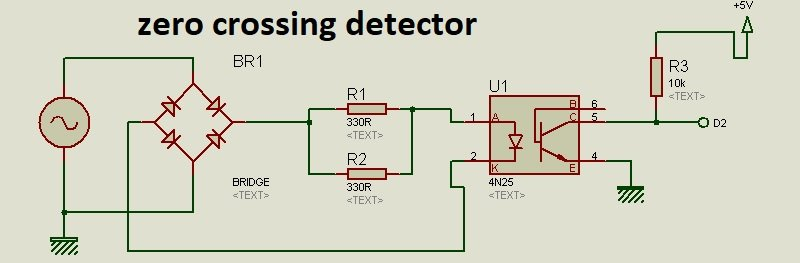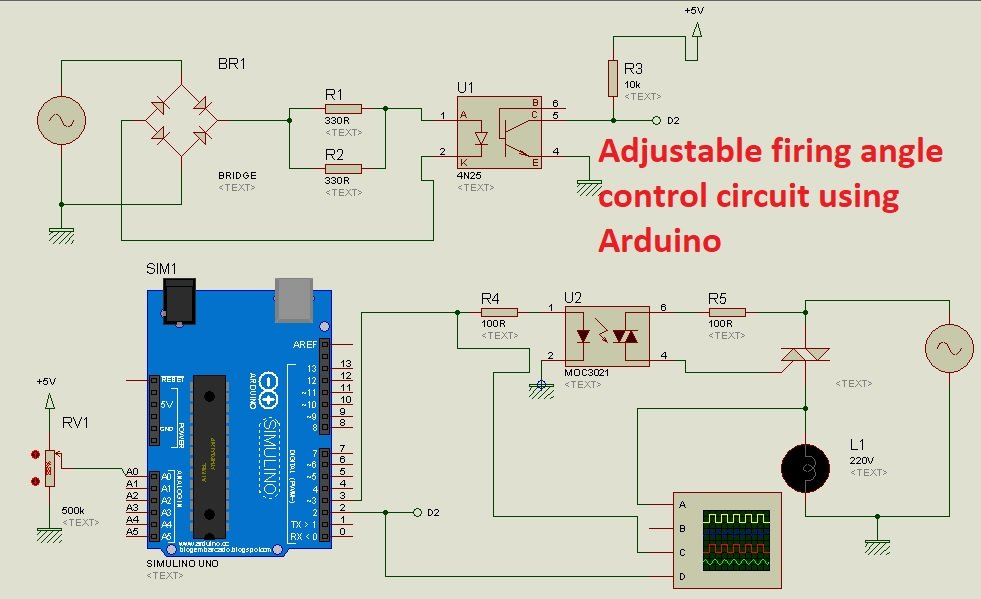# adjustable firing angle control of thyristor using arduino

Adjustable firing angle control of thyristor using arduino, Hi everyone this project is about adjustable firing angle control of triac or thyristor using Arduino Uno. In this project , we have designed a circuit diagram to control the phase angle of thyristor over a complete sine wave or complete ac cycle for both negative and positive half cycle. This project has many applications like ac power control with thyristor and speed control of single phase induction motor.I have already posted a article on thyristor firing angle control circuit using analog electronics components. But in this project, I have created a adjustable firing angle with digital electronics components like Arduino.  So now lets start with basic introduction of all the components which are used in this adjustable phase angle control of thyristor using arduino.

### Components  used in adjustable firing angle control of thyristor

Followings are the main components used in this project.

• Zero crossing detection circuit
• Bride rectifier : It is used to convert negative half cycle to positive half cycle.
• resistors
• 4N25 optocoupler
• diodes
• Thyristor
• Arduino Uno R3
• variable resistor : It is used to control firing angle time.

### Zero crossing detection circuit

Circuit diagram of zero crossing detection circuit is shown below.  Zero crossing circuit is used to detect zero crossing of ac cycle after every half cycle. Because we want to control phase angle for both positive and negative half cycles. The length of triggering pulse should be same for both positive and negative half cycle. So zero crossing detector circuit is must to design adjustable firing angle control of thyristor using arduino. 4N25 optocoupler is used for isolation between high side voltage and low side voltage and it also helps to detect zero crossing. The output of zero crossing D2 will be fed to Arduino.### Circuit diagram of adjustable firing angle control of thyristor using arduino

• Complete circuit diagram of adjustable firing angle control of thyristor using arduino  is shown below.
• In this circuit diagram variable resistor is connected with analog channel zero of Arduino.
• Variable resistor is used to adjust the firing angle of thyristor or triac.
• MOC3021  is a optically isolated thyristor or triac driver.
• Input to MOC3021 is firing pulse for thyristor which is used to turn on and turn off thyristor.
• At the output 220V AC lamp is used in series of ac power supply.
• When thyristor is off, lamp will be off and when thryistor is on, lamp will also be on.
• So the power across the lamp depends on the on time of thyristor which is ultimately depends on firing angle of thyristorYou may also like to check other similar article:

### 10 thoughts on “adjustable firing angle control of thyristor using arduino”

1. please post code in C language illustrate how to AVR Atmega control SCR

2. Gửi cho tôi mã điều khiển góc mở thyristor bằng adruino.

3. Hi, can you send me the code please?

4. i need code plz sir contect me

5. Can u provide me code of this program?

6. hi
very thanks

I have two questions:

1- when using an optocoupler, can it be used for many years?
(in this case, can the optocoupler retain its characteristics for many years?!)

2-isn’t it better to put current limit resistor before bridge diode?
in this case, only one terminal has a high voltage.( one head of current limiting resistor)

7. What Wattage resistors for R1 & R2 are you using?
Surely, they’re going to get hot!